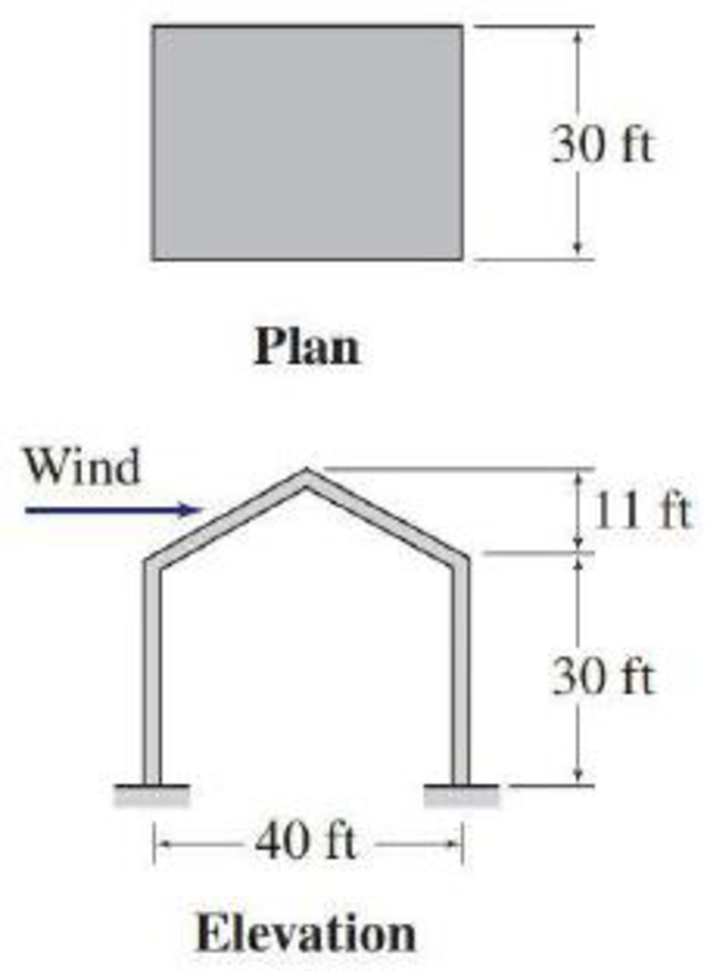# Determine the external wind pressures on the windward and leeward walls of the building of Problem 2.14. See Fig. P2.14. 2.14 Determine the external wind pressure on the roof of the rigid-gabled frame of a building for an essential disaster operation center shown in Fig. P2.14. The building is located in Kansas City, Missouri, where the terrain is representative of exposure C and the basic wind speed for risk category IV buildings is 120 mph. Assume the wind direction is normal to the ridge, as shown in the figure. Use K d = 0.85 for main wind-force resisting systems, and K e = 1.

#### Solutions

Chapter
Section
Chapter 2, Problem 15P
Textbook Problem
170 views

## Determine the external wind pressures on the windward and leeward walls of the building of Problem 2.14. See Fig. P2.14.2.14 Determine the external wind pressure on the roof of the rigid-gabled frame of a building for an essential disaster operation center shown in Fig. P2.14. The building is located in Kansas City, Missouri, where the terrain is representative of exposure C and the basic wind speed for risk category IV buildings is 120 mph. Assume the wind direction is normal to the ridge, as shown in the figure. Use Kd = 0.85 for main wind-force resisting systems, and Ke = 1.

To determine

Find the external wind pressure acting on the windward and leeward walls of the building.

### Explanation of Solution

Given information:

The terrain of the city belongs to Exposure C.

The building belongs to risk category IV.

The basic wind speed is V=120mph.

The direction of the wind is normal to the ridge.

Calculation:

Show the sloped roof of the building as shown in Figure 1.

Refer Figure 1.

The length and width of the building are L=40ft and B=30ft.

Calculate the ratio LB as follows:

LB=40ft30ft=1.33

Calculate the angle of slope (θ) of the roof using the relation:

tanθ=11ft20ftθ=tan1(0.55)θ=28.8°

Calculate the mean roof height (h) as follows:

h=30ft+11ft2=35.5ft

Refer the Table 2.4 “Exposure Categories for Buildings for Wind Loads” in the textbook.

For exposure C.

The values of gradient height is zg=900ft.

The value of power law coefficient is α=9.5.

Show the expression for the velocity pressure coefficient (Kh) as follows:

Kh=2.01(hzg)2α (1)

Substitute 35.5ft for h, 900ft for zg, and 9.5 for α in Equation (1).

Kh=2.01(35.5900)29.5=2.01×0.50631=1.0171.02

The value of the topographic factor (Kzt) as 1 for main wind force resisting system.

The value of the wind directionality factor (Kd) is 0.85for main wind force resisting system.

The value of the ground elevation factor (Ke) is 1 for ground elevation at sea level.

Show the expression for the velocity pressure (qh) at height h as follows:

qh=0.00256KhKztKdKeV2 (2)

Here, qh is in pounds per square foot.

Substitute 1.02 for Kh, 1 for Kzt, 0.85 for Kd, 1 for Ke, and 120mph for V in Equation (2).

qh=0.00256×1.02×1×0.85×1×1202=31.961psf32psf

Take the value of the gust effect factor (G) as 0.85 for rigid structures.

Consider Leeward Side of the wall.

Refer Figure 2.12 “External Pressure Coefficients, Cp, for Loads on Main Wind-Force

Resisting Systems for Enclosed or Partially Enclosed Buildings of All Heights” in the text book.

The wall pressure coefficient is Cp=0.5 for LB=1.

The wall pressure coefficient is Cp=0.3 for LB=2.

Find the value of wall pressure coefficient Cp for LB=1.33

Cp(0.5)0.3(0.5)=1.33121Cp+0.5=0.33×0.2Cp=0.0660.5Cp=0.434

Cp0.45

Calculate the wind pressure (ph) using the relation:

ph=qhGCp (3)

Substitute 32psf for qh, 0

### Still sussing out bartleby?

Check out a sample textbook solution.

See a sample solution

#### The Solution to Your Study Problems

Bartleby provides explanations to thousands of textbook problems written by our experts, many with advanced degrees!

Get Started

Find more solutions based on key concepts
Determine the mass moment of inertia of the earth about its axis of rotation, going through the poles. Assume t...

Engineering Fundamentals: An Introduction to Engineering (MindTap Course List)

Describe and characterize the skills desired for a DBA.

Database Systems: Design, Implementation, & Management

Define a class, subclass, and superclass, and provide three examples of each.

Systems Analysis and Design (Shelly Cashman Series) (MindTap Course List)

Describe the role of a corporate trainer.

Enhanced Discovering Computers 2017 (Shelly Cashman Series) (MindTap Course List)

What does GDT stand for?

Precision Machining Technology (MindTap Course List)

What is Port Address Translation (PAT) and how does it work?

Principles of Information Security (MindTap Course List)

If your motherboard supports ECC DDR3 memory, can you substitute non-ECC DDR3 memory?

A+ Guide to Hardware (Standalone Book) (MindTap Course List)

What effect does the root opening or root gap have on a butt joint?

Welding: Principles and Applications (MindTap Course List)